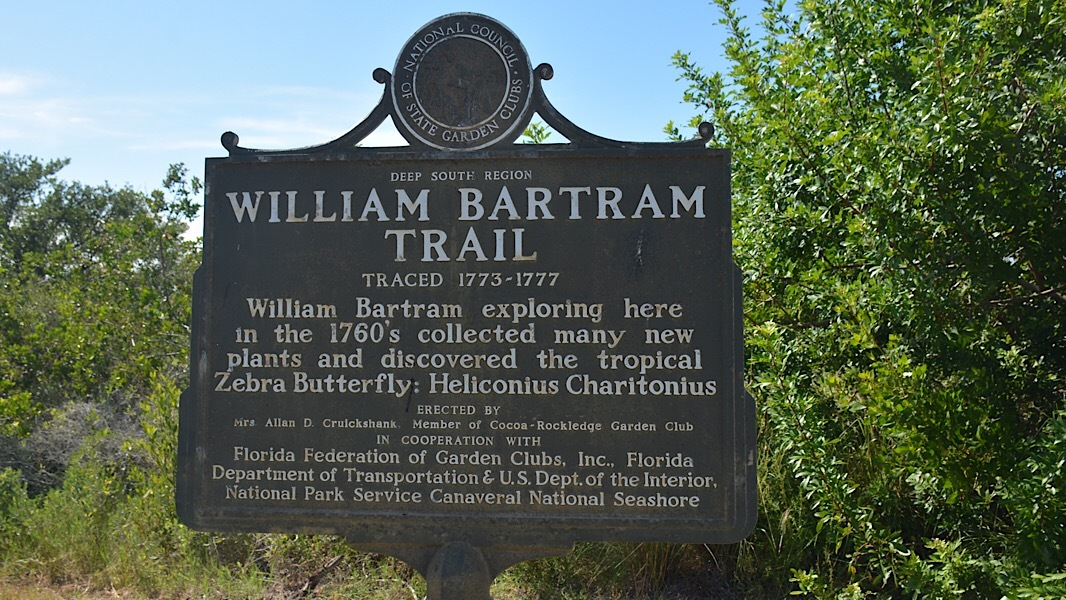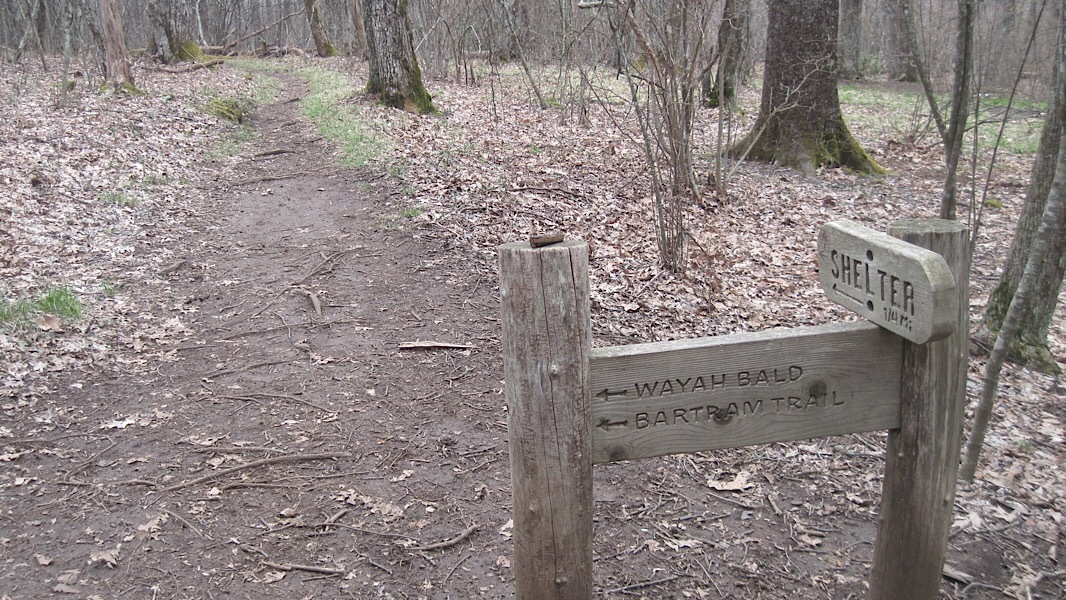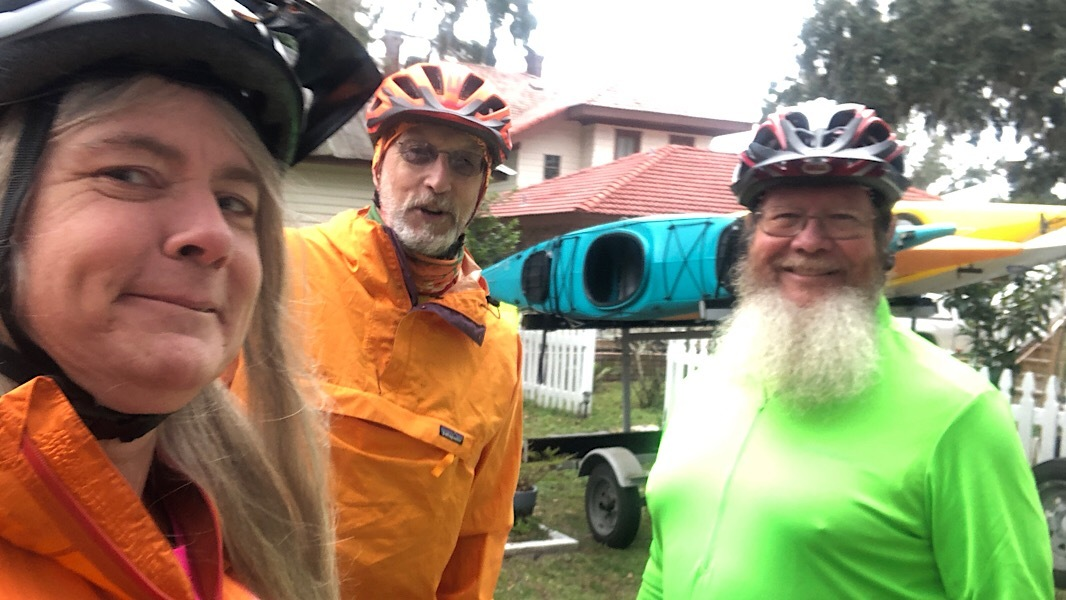Like   Tweet   Pin   +1   in

## Following the Bartram TrailBartram Trail sign at Playalinda Beach

When we first encountered the Bartram Trail, it was as a series of state historic markers scattered across Florida. We'd seen them at places like Paynes Prairie, Manatee Springs, and Mead Gardens, and knew they were connected to William Bartram, a naturalist who, in 1773 and 1774, explored Florida on foot and by canoe. Two centuries after his journey, the Florida Federation of Garden Clubs started placing these markers in key spots across the state. During the Bicentennial, a Bartram Trail Conference was established to help research and site these markers, and to grow public interest about Bartram's Travels.Bartram Trail intersection near Wayah Bald Shelter on the AT

Two decades later, we discovered a Bartram Trail that connected to the Appalachian Trail. Paralleling the AT, it's a long distance hiking trail stretching 116 miles through the mountains of Georgia and North Carolina, roughly paralleling the AT. We first learned about it from friends who hiked it, and then ran across intersections with it ourselves near Wayah Bald and Cheoah Bald. The folks we know who've hiked it say it's much tougher terrain than the AT, lightly used and maintained, and wonderfully wild. Swami has a nice writeup about it here.With local guides, we hiked, biked, and paddled the Bartram Trail in Florida

Two summers ago, we were driving through Palatka when we saw a sign for the St. Johns River Center and decided to stop and see what it was all about. Good thing. For right in front of the door was a "Bartram National Recreation Trail" sign in the trademark shape used by all National Trails Systems trails. That started us on a quest to find out what this new trail in Florida was about. Dig into our articles below and follow along.

Cheers, Sandra & John

PS. Headed to Appalachian Trail Days? We plan to bust some myths about the Florida Trail with a contrast and compare workshop - AT vs. FT - at "Hiking the Florida Trail," 10-11:30 AM on Saturday May 18 at the Old Mill. Join us! Details here.

 table div table+table+table+table+table+table div table{width:100%;padding:0}table div table+table+table+table+table+table div table img{width:96.23%;padding:0;float:none}table div table+table+table+table+table+table div table td{width:100%;padding:0 1.88% 18px}/* styles */## The Bartram Trail in Putnam County

 table div table+table+table+table+table+table+table+table div table,table.module-7{width:41.13%;float:left;padding:0}table div table+table+table+table+table+table+table+table div table a{border:0 none;text-decoration:none}table div table+table+table+table+table+table+table+table div table img{width:100%!important;border:0 none;text-decoration:none}table div table+table+table+table+table+table+table+table div table td{width:100%;padding:0 20px 20px 0}/* styles */ On a Bartram Adventure Tour On a guided Bartram Adventure Tour, we hiked, biked, and paddled to follow the travels of William and John Bartram along the St. Johns River on the newly designated Bartram National Recreation Trail, centered in Palatka, Florida.

## HIKE * BIKE * PADDLE * RELAX

 table div table+table+table+table+table+table+table+table+table+table div table,table.module-9{width:41.13%;float:left;padding:0}table div table+table+table+table+table+table+table+table+table+table div table a{border:0 none;text-decoration:none}table div table+table+table+table+table+table+table+table+table+table div table img{width:100%!important;border:0 none;text-decoration:none}table div table+table+table+table+table+table+table+table+table+table div table td{width:100%;padding:0 20px 20px 0}/* styles */ William Bartram St. Johns River Loop Discover the beauty of Welaka State Forest on a 5 mile footpath that winds along ecotones and hugs the bluffs of the St. Johns River to showcase the natural wonders that William Bartram documented in 1774.
 table div table+table+table+table+table+table+table+table+table+table+table div table,table.module-10{width:41.51%;float:left;padding:0}table div table+table+table+table+table+table+table+table+table+table+table div table a{border:0 none;text-decoration:none}table div table+table+table+table+table+table+table+table+table+table+table div table img{width:100%!important;border:0 none;text-decoration:none}table div table+table+table+table+table+table+table+table+table+table+table div table td{width:100%;padding:0 20px 20px 0}/* styles */ Palatka-Lake Butler Trail With a corridor spanning nearly 50 miles through North Florida, the Palatka-Lake Butler State Trail provides paved trail for riders and an unpaved corridor used by the Florida Trail.
 table div table+table+table+table+table+table+table+table+table+table+table+table div table,table.module-11{width:41.32%;float:left;padding:0}table div table+table+table+table+table+table+table+table+table+table+table+table div table a{border:0 none;text-decoration:none}table div table+table+table+table+table+table+table+table+table+table+table+table div table img{width:100%!important;border:0 none;text-decoration:none}table div table+table+table+table+table+table+table+table+table+table+table+table div table td{width:100%;padding:0 20px 20px 0}/* styles */ Paddling the Bartram Trail in Welaka Learning how William and John Bartram traveled along the St. Johns River in the 1700s, I followed an experienced river guide for a look at part of the Bartram National Recreation Trail from the water on our Bartram Adventure Tour.
 table div table+table+table+table+table+table+table+table+table+table+table+table+table div table,table.module-12{width:41.32%;float:left;padding:0}table div table+table+table+table+table+table+table+table+table+table+table+table+table div table a{border:0 none;text-decoration:none}table div table+table+table+table+table+table+table+table+table+table+table+table+table div table img{width:100%!important;border:0 none;text-decoration:none}table div table+table+table+table+table+table+table+table+table+table+table+table+table div table td{width:100%;padding:0 20px 20px 0}/* styles */ Palatka Riverfront Park Recently re-imagined to showcase the beauty of the St. Johns River, Palatka Riverfront Park offers a quarter-mile riverfront walk with panoramic views
 /* styles */ This region is covered in our book Explorer’s Guide: North Florida & The Panhandle, an excellent companion for planning a trip to the Palatka area.
 table div table+table+table+table+table+table+table+table+table+table+table+table+table+table+table+table div table{width:100%;padding:0}table div table+table+table+table+table+table+table+table+table+table+table+table+table+table+table+table div table img{width:96.23%;padding:0;float:none}table div table+table+table+table+table+table+table+table+table+table+table+table+table+table+table+table div table td{width:100%;padding:0 1.88% 18px}/* styles */## Recent Features

 table div table+table+table+table+table+table+table+table+table+table+table+table+table+table+table+table+table+table div table,table.module-17{width:37.55%;float:left;padding:0}table div table+table+table+table+table+table+table+table+table+table+table+table+table+table+table+table+table+table div table a{border:0 none;text-decoration:none}table div table+table+table+table+table+table+table+table+table+table+table+table+table+table+table+table+table+table div table img{width:100%!important;border:0 none;text-decoration:none}table div table+table+table+table+table+table+table+table+table+table+table+table+table+table+table+table+table+table div table td{width:100%;padding:0 20px 20px 0}/* styles */ Blueberries Ever After It’s blueberry season in Florida! April is the month when it’s time to visit a commercial grower and start picking. We did just that at our local berry farm, Ever After Farms.
 table div table+table+table+table+table+table+table+table+table+table+table+table+table+table+table+table+table+table+table div table,table.module-18{width:38.11%;float:left;padding:0}table div table+table+table+table+table+table+table+table+table+table+table+table+table+table+table+table+table+table+table div table a{border:0 none;text-decoration:none}table div table+table+table+table+table+table+table+table+table+table+table+table+table+table+table+table+table+table+table div table img{width:100%!important;border:0 none;text-decoration:none}table div table+table+table+table+table+table+table+table+table+table+table+table+table+table+table+table+table+table+table div table td{width:100%;padding:0 20px 20px 0}/* styles */ Identifying Birds in Florida Florida is a prime destination for migratory birds. Here is identification information for some of the bird species you’ll see in Florida almost any time of year.
 table div table+table+table+table+table+table+table+table+table+table+table+table+table+table+table+table+table+table+table+table div table,table.module-19{width:38.49%;float:left;padding:0}table div table+table+table+table+table+table+table+table+table+table+table+table+table+table+table+table+table+table+table+table div table a{border:0 none;text-decoration:none}table div table+table+table+table+table+table+table+table+table+table+table+table+table+table+table+table+table+table+table+table div table img{width:100%!important;border:0 none;text-decoration:none}table div table+table+table+table+table+table+table+table+table+table+table+table+table+table+table+table+table+table+table+table div table td{width:100%;padding:0 20px 20px 0}/* styles */ The Trans-Florida Central Railroad Trail If you’ve seen the prominent Trans-Florida Central Railroad Trail over Interstate 95 at Fellsmere, you’ve probably wondered about it. Here’s what we found when we stopped for a ride.
 table div table+table+table+table+table+table+table+table+table+table+table+table+table+table+table+table+table+table+table+table+table div table,table.module-20{width:37.74%;float:left;padding:0}table div table+table+table+table+table+table+table+table+table+table+table+table+table+table+table+table+table+table+table+table+table div table a{border:0 none;text-decoration:none}table div table+table+table+table+table+table+table+table+table+table+table+table+table+table+table+table+table+table+table+table+table div table img{width:100%!important;border:0 none;text-decoration:none}table div table+table+table+table+table+table+table+table+table+table+table+table+table+table+table+table+table+table+table+table+table div table td{width:100%;padding:0 20px 20px 0}/* styles */ Sams House at Pine Island A delightful immersion into the natural side of Merritt Island, the 2.8 miles of trails at Sams House at Pine Island range from mild to wild as they draw you deeper into coastal habitats along the Indian River Lagoon.
 table div table+table+table+table+table+table+table+table+table+table+table+table+table+table+table+table+table+table+table+table+table+table div table{width:100%;padding:0}table div table+table+table+table+table+table+table+table+table+table+table+table+table+table+table+table+table+table+table+table+table+table div table img{width:96.23%;padding:0;float:none}table div table+table+table+table+table+table+table+table+table+table+table+table+table+table+table+table+table+table+table+table+table+table div table td{width:100%;padding:0 1.88% 18px}/* styles */## Our Public Workshops

May 18: Hiking the Florida Trail, Appalachian Trail Days, Damascus VA

## Outdoor Events

Are we missing any major events? Let us know and we'll add them to the next newsletter.

 table div table+table+table+table+table+table+table+table+table+table+table+table+table+table+table+table+table+table+table+table+table+table+table+table+table div table{width:100%;padding:0}table div table+table+table+table+table+table+table+table+table+table+table+table+table+table+table+table+table+table+table+table+table+table+table+table+table div table img{width:96.23%;padding:0;float:none}table div table+table+table+table+table+table+table+table+table+table+table+table+table+table+table+table+table+table+table+table+table+table+table+table+table div table td{width:100%;padding:0 1.88% 18px}/* styles *//* styles */